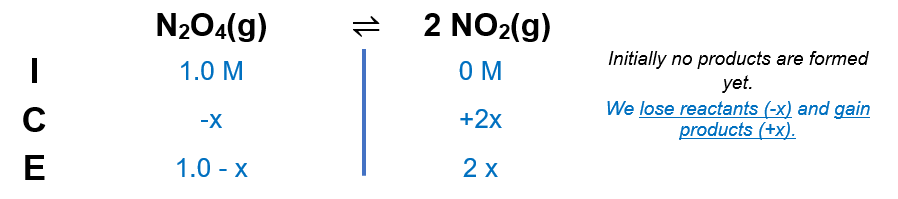# Problem: Exactly 1.0 mol N2O4 is placed in an empty 1.0-L container and allowed to reach equilibrium described by the equationN2O4(g) ⇌ 2NO2(g).If at equilibrium N2O4 is 28.0% dissociated, what is the value of the equilibrium constant, Kc, for the reaction under these conditions?A) 0.44B) 2.3C) 0.31D) 0.78E) 0.11

###### FREE Expert Solution

We’re being asked to calculate the equilibrium constant of HA if a 0.0538 M solution is 3.57% ionized.

Recall that the percent ionization is given by:

The dissociation is as follows:

N2O4(g) ⇌ 2NO2(g)

From this, we can construct an ICE table. Remember that liquids are ignored in the ICE table and Ka expression.The Kc expression for is:

$\overline{){{\mathbf{K}}}_{{\mathbf{c}}}{\mathbf{=}}\frac{\mathbf{products}}{\mathbf{reactants}}{\mathbf{=}}\frac{{\mathbf{\left[}{\mathbf{NO}}_{\mathbf{2}}\mathbf{\right]}}^{\mathbf{2}}}{\mathbf{\left[}{\mathbf{N}}_{\mathbf{2}}{\mathbf{O}}_{\mathbf{4}}\mathbf{\right]}}}$

Note that each concentration is raised by the stoichiometric coefficient: [NO2] is raised to 2 and [N2O4] is raised to 1.

94% (227 ratings)###### Problem Details

Exactly 1.0 mol N2O4 is placed in an empty 1.0-L container and allowed to reach equilibrium described by the equation

N2O4(g) ⇌ 2NO2(g).

If at equilibrium N2O4 is 28.0% dissociated, what is the value of the equilibrium constant, Kc, for the reaction under these conditions?

A) 0.44

B) 2.3

C) 0.31

D) 0.78

E) 0.11